In :
#format the book
%matplotlib inline
from __future__ import division, print_function
import book_format

Out:

In the last chapter we discussed the difficulties that nonlinear systems pose. This nonlinearity can appear in two places. It can be in our measurements, such as a radar that is measuring the slant range to an object. Slant range requires you to take a square root to compute the x,y coordinates:

$$x=\sqrt{slant^2 - altitude^2}$$

The nonlinearity can also occur in the process model - we may be tracking a ball traveling through the air, where the effects of gravity and air drag lead to highly nonlinear behavior. The standard Kalman filter performs poorly or not at all with these sorts of problems.

In the last chapter I showed you a plot like this. I have altered the equation somewhat to emphasize the effects of nonlinearity.

In :
from numpy.random import normal
import numpy as np
import matplotlib.pyplot as plt
from nonlinear_plots import plot_nonlinear_func

gaussian=(0., 1.)
data = normal(loc=gaussian, scale=gaussian, size=500000)

def g(x):
return (np.cos(4*(x/2 + 0.7))) - 1.3*x

with book_format.figsize(y=5):
plot_nonlinear_func(data, g, gaussian=gaussian)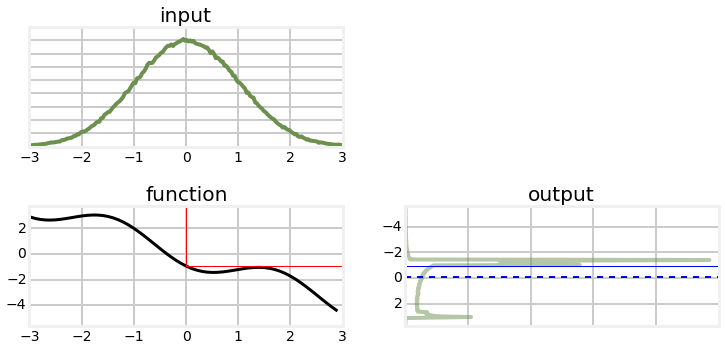I generated this by taking 500,000 samples from the input, passing it through the nonlinear transform, and building a histogram of the result. We call these points sigma points. From the output histogram we can compute a mean and standard deviation which would give us an updated, albeit approximated Gaussian.

It has perhaps occurred to you that this sampling process constitutes a solution to our problem. Suppose for every update we generated 500,000 points, passed them through the function, and then computed the mean and variance of the result. This is called a 'Monte Carlo' approach, and it used by some Kalman filter designs, such as the Ensemble filter and particle filter. Sampling requires no specialized knowledge, and does not require a closed form solution. No matter how nonlinear or poorly behaved the function is, as long as we sample with enough sigma points we will build an accurate output distribution.

"Enough points" is the rub. The graph above was created with 500,000 sigma points, and the output is still not smooth. What's worse, this is only for 1 dimension. In general, the number of points required increases by the power of the number of dimensions. If you only needed 500 points for 1 dimension, you'd need 500 squared, or 250,000 points for two dimensions, 500 cubed, or 125,000,000 points for three dimensions, and so on. So while this approach does work, it is very computationally expensive. The Unscented Kalman filter uses a similar technique but reduces the amount of computation needed by a drastic amount by using a deterministic method of choosing the points.

## Sigma Points - Sampling from the Distribution¶

Let's look at the problem in terms of a 2D covariance ellipse. I choose 2D merely because it is easy to plot; this will extend to any number of dimensions. Assuming some arbitrary nonlinear function, we will take random points from the first covariance ellipse, pass them through the nonlinear function, and plot their new position. Then we can compute the the mean and covariance of the transformed points, and use that as our estimate of the mean and probability distribution.

In :
import ukf_internal
ukf_internal.show_2d_transform()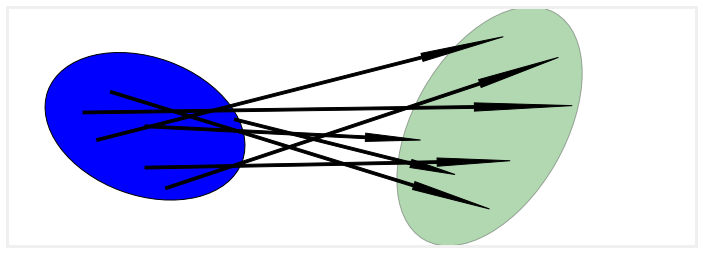On the left we show an ellipse depicting the $1\sigma$ distribution of two state variables. The arrows show how several randomly sampled points might be transformed by some arbitrary nonlinear function to a new distribution. The ellipse on the right is drawn semi-transparently to indicate that it is an estimate of the mean and variance of this collection of points - if we were to sample, say, a million points the shape of the points might be very far different than an ellipse.

Let's look at that by running a bunch of points through a nonlinear function. We will write a function to pass randomly generated points with a Gaussian distribution through the system

\begin{aligned}x&=x+y\\ y &= 0.1x^2 + y^2\end{aligned}

for the mean and covariance

$$\mu = \begin{bmatrix}0\\0\end{bmatrix}, \Sigma=\begin{bmatrix}32&15\\15&40\end{bmatrix}$$
In :
import numpy as np
from numpy.random import multivariate_normal
from nonlinear_plots import plot_monte_carlo_mean

def f(x,y):
return x+y, .1*x**2 + y*y

mean = (0, 0)
p = np.array([[32, 15], [15., 40.]])

# Compute linearized mean
mean_fx = f(*mean)

#generate random points
xs, ys = multivariate_normal(mean=mean, cov=p, size=10000).T
plot_monte_carlo_mean(xs, ys, f, mean_fx, 'Linearized Mean');

Difference in mean x=-0.022, y=43.053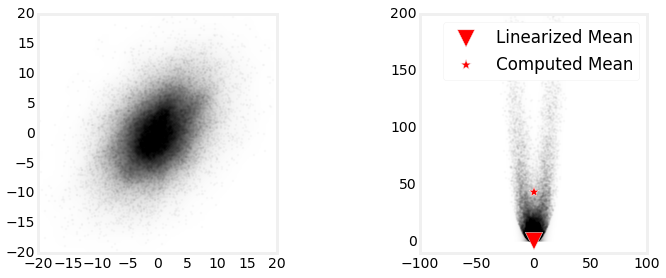This plot shows the strong nonlinearity that occurs with this function, and the large error that would result if we linearized the function at (0,0), which is what filters like the Extended Kalman filters do (we will be learning this in the next chapter).

## Choosing Sigma Points¶

I used 10,000 randomly selected sigma points to generate this solution. While the computed mean is quite accurate, computing 10,000 points for every update would cause our filter to be very slow. So, what would be fewest number of sampled points that we can use, and what kinds of constraints does this problem formulation put on the points? We will assume that we have no special knowledge about the nonlinear function as we want to find a generalized algorithm that works for any function. We can visualize this algorithm using the following diagram.

In :
ukf_internal.show_sigma_transform()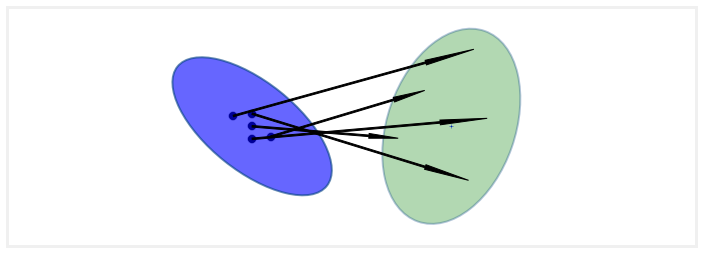Let's consider the simplest possible case and see if it offers any insight. The simplest possible system is the identity function. In mathematical notation this is $f(x) = x$. It should be clear that if our algorithm does not work for the identity function then the filter will never converge. In other words, if the input is 1 (for a one dimensional system), the output must also be 1. If the output was different, such as 1.1, then when we fed 1.1 into the transform at the next time step, we'd get out yet another number, maybe 1.23. The filter would run away (diverge).

The fewest number of points that we can use is one per dimension. This is the number that the linear Kalman filter uses. The input to a Kalman filter for the distribution $\mathcal{N}(\mu,\sigma^2)$ is $\mu$ itself. So while this works for the linear case, it is not a good answer for the nonlinear case.

Perhaps we can use one point per dimension, but altered somehow. However, if we were to pass some value $\mu+\Delta$ into the identity function $f(x)=x$ it would not converge, so this is not a possible algorithm. We must conclude that a one point sample will not work.

So, what is the next lowest number we can choose? Consider the fact that Gaussians are symmetric, and that we probably want to always have one of our sample points be the mean of the input for the identity function to work. Two points would require us to select the mean, and then one other point. That one other point would introduce an asymmetry in our input that we probably don't want. It would be very difficult to make this work for the identity function $f(x)=x$.

The next lowest number is 3 points. 3 points allows us to select the mean, and then one point on each side of the mean, as depicted on the chart below.

In :
ukf_internal.show_3_sigma_points()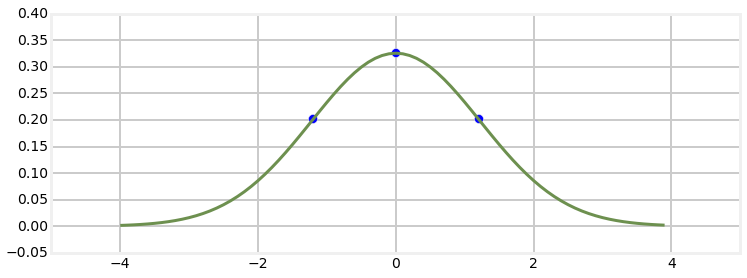So we can take those three points, pass them through a nonlinear function f(x), and compute a new mean and variance. We can compute the mean as the average of the 3 points, but that is not very general. For example, for a very nonlinear problem we might want to weight the center point much higher than the outside points, or we might want to weight the outside points higher if the distribution is not Gaussian. A more general approach is to compute the mean as $\mu = \sum_i w_if(\mathcal{X}_i)$.

For this to work for identity we will want the sums of the weights for the mean to equal one. We can always come up with counterexamples, but in general if the sum is greater or less than one the sampling will not yield the correct output. Given that, we then have to select sigma points $\mathcal{X}$ and their corresponding weights so that they compute to the mean and variance of the input Gaussian. It is possible to use different weights for the mean ($w^m$) and for the variance ($w^c$). So we can write

\begin{aligned} \mathbf{Constraints:}\\ \sum_i{w_i^m}&=1 \\ \mu &= \sum_i w_i^mf(\mathcal{X}_i) \\ \Sigma &= \sum_i w_i^c{(f(\mathcal{X})_i-\mu)(f(\mathcal{X})_i-\mu)^\mathsf{T}} \end{aligned}

If we look at this is should be clear that there is no one unique answer - the problem is unconstrained. For example, if you choose a smaller weight for the point at the mean for the input, you could compensate by choosing larger weights for the rest of the $\mathcal{X}$, and vice versa. We can use different weights for the mean and variances, or the same weights. Indeed, these equations do not require that any of the points be the mean of the input at all, though it seems 'nice' to do so, so to speak.

But before we go on I want to make sure the idea is clear. We are choosing 3 points for each dimension in our covariances. That choice is entirely deterministic. Below are three different examples for the same covariance ellipse.

In :
ukf_internal.show_sigma_selections()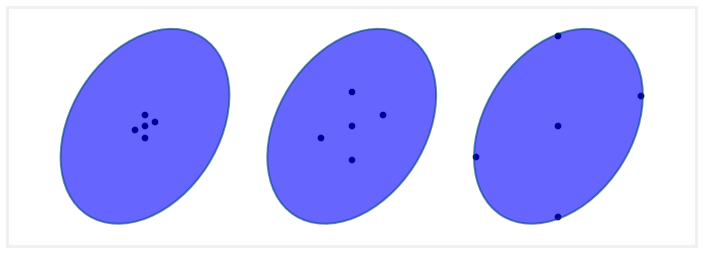The points do not lie along the major and minor axis of the ellipse; nothing in the constraints above require me to do that. Furthermore, in each case I show the points evenly spaced; again, the constraints above do not require that.

We can see that the arrangement and spacing of the sigma points will affect how we sample our distribution. Points that are close together will sample local effects, and thus probably work better for very nonlinear problems. Points that are far apart, or far off the axis of the ellipse will sample non-local effects and non Gaussian behavior. However, by varying the weights used for each point we can mitigate this. If the points are far from the mean but weighted very slightly we will incorporate some of the knowledge about the distribution without allowing the nonlinearity of the problem to create a bad estimate.

## Van der Merwe's Scaled Sigma Point Algorithm¶

There are several published ways for selecting the sigma points for the Unscented Kalman filter, and you are free to invent your own. However, since 2005 or so research and industry have mostly settled on the version published by Rudolph Van der Merwe in his 2004 PhD dissertation  because it performs well with a variety of problems and it has a good tradeoff between performance and accuracy. It is a slight reformulation of the Scaled Unscented Transform published by Simon J. Julier .

Before we work through the derivation, let's look at an example. I will plot the sigma points on top of a covariance ellipse showing the first and second standard deviations, and size them based on the weights assigned to them.

In :
ukf_internal.plot_sigma_points()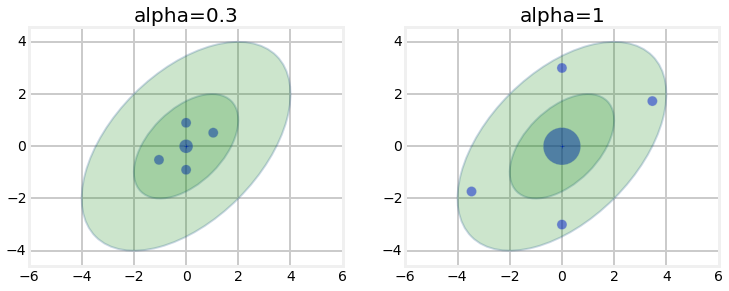3.91


We can see that the sigma points lie between the first and second deviation, and that the larger $\alpha$ spreads the points out further. Furthermore, the larger $\alpha$ weighs the mean (center point) higher than the smaller $\alpha$, and weighs the rest of the sigma points less. This should fit our intuition - the further a point is from the mean the less we should weight it. We don't know how these weights and sigma points are selected yet, but the choices look reasonable.

Van der Merwe's formulation uses 3 parameters to control how the sigma points are distributed and weighted: $\alpha$, $\beta$, and $\kappa$. We will go into detail with these later. For now, $\beta=2$ is a good choice for Gaussian problems, $\kappa=3-N$ is a good choice for $\kappa$, and $0 \le \alpha \le 1$ is an appropriate choice for $\alpha$, where a larger value spreads the sigma points further from the mean.

Our first sigma point is always going to be the mean of our input. This is the sigma point displayed in the center of the ellipses in the diagram above. We will call this $\boldsymbol{\chi}_0$. So,

$$\mathcal{X}_0 = \mu$$

For notational convenience we define $\lambda = \alpha^2(n+\kappa)-n$. Then the remaining sigma points are computed as

\begin{aligned} \boldsymbol{\chi}_i &= \mu + (\sqrt{(n+\lambda)\Sigma})_i\,\,\,\, &\text{for}\text{ i=1 .. n} \\ \boldsymbol{\chi}_i &= \mu - (\sqrt{(n+\lambda)\Sigma})_{i-n}\,\,\,\,\, &\text{for}\text{ i=(n+1) .. 2n} \\ \end{aligned}

In other words, we scale the covariance matrix by a constant, take the square root of it, and then to ensure symmetry both add and subtract if from the mean. We will discuss how you take the square root of a matrix later.

Van der Merwe's forumation uses one set of weights for the means, and another set for the covariance. The weights for the mean of $\mathcal{X}_0$ is computed as

$$W^m_0 = \frac{\lambda}{n+\lambda}$$

The weight for the covariance of $\mathcal{X}_0$ is

$$W^c_0 = \frac{\lambda}{n+\lambda} + 1 -\alpha^2 + \beta$$

The weights for the rest of the sigma points $\boldsymbol{\chi}_1 ... \boldsymbol{\chi}_{2n}$ are the same for the mean and covariance. They are

$$W^m_i = W^c_i = \frac{1}{2(n+\lambda)}\;\;\;i=1..2n$$

It may not be obvious why this is 'correct', and indeed, it cannot be proven that this is ideal for all nonlinear problems. But you can see that we are choosing the sigma points proportional to the square root of the covariance matrix, and the square root of variance is standard deviation. So, the sigma points are spread roughly according to 1 standard deviation. However, there is an $n$ term in there - the more dimensions there are the more the points will be spread out and weighed less.

The update step converts the sigmas into measurement space via the h(x) function.

$$\mathcal{Z} = h(\mathcal{Y})$$

The mean and covariance of those points is computed with the unscented transform. The residual and Kalman gain is then computed. The cross variance is computed as:

$$\mathbf{P}_{xz} =\sum W(\mathcal{X}-x)(\mathcal{X_z}-\mathbf{x}_z)^\mathsf{T}$$

Finally, we compute the new state estimate using the residual and Kalman gain:

$$\hat{\mathbf{x}} = \mathbf{x}^- + \mathbf{Ky}$$

and the new covariance is computed as:

$$\mathbf{P} = \mathbf{P}^- - \mathbf{PKP}_z\mathbf{K}^\mathsf{T}$$

This function can be implemented as follows, assuming it is a method of a class that stores the necessary matrices and data.

The computation of the new mean and covariance is called the unscented transform.

## The Unscented Transform¶

The unscented transform is the core of the algorithm yet it is remarkably simple. For each dimension in the state space we deterministically choose 3 sigma points and corresponding weights using the algorithm above. We pass the sigma points through the nonlinear function yielding a transformed set of points

$$\boldsymbol{\mathcal{Y}} = f(\boldsymbol{\chi})$$
In :
ukf_internal.show_sigma_transform(with_text=True)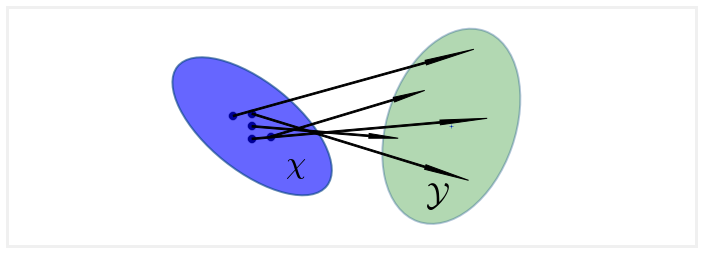We then compute the new mean and covariance using these equations.

\begin{aligned} \mu &= \sum_i w_i\boldsymbol{\mathcal{Y}}_i \\ \Sigma &= \sum_i w_i{(\boldsymbol{\mathcal{Y}}_i-\mu)(\boldsymbol{\mathcal{Y}}_i-\mu)^\mathsf{T}} \end{aligned}

These equations should be familar - they are the constraint equations we developed above. They perhaps don't look like much, but let's see an example of their power.

Earlier we wrote a function that found the mean of a distribution by passing 50,000 points through a nonlinear function. Let's now use 5 sigma points - we will pass them through the nonlinear function, and compute their mean with the unscented transform. This code is below. Under the comment ### create sigma points I use code from FilterPy to generate the sigma points. It uses functionality which you will learn later in this chapter; pass by it for now. The key points are we generate 5 points deterministically, pass them through the nonlinear function, and then run the unscented transform on them to generate the estimated mean.

In :
from filterpy.kalman import unscented_transform, MerweScaledSigmaPoints
import scipy.stats as stats

def f_nonlinear_xy(x, y):
return np.array([x + y, .1*x**2 + y*y])

mean = (0, 0)
p = np.array([[32., 15],
[15., 40.]])

### create sigma points
points = MerweScaledSigmaPoints(n=2, alpha=.1, beta=2., kappa=1.)
Wm, Wc = points.weights()
sigmas = points.sigma_points(mean, p)

### pass through nonlinear function
sigmas_f = np.zeros((5, 2))
for i in range(5):
sigmas_f[i] = f_nonlinear_xy(sigmas[i, 0], sigmas[i ,1])

### pass through unscented transform
ukf_mean, _ = unscented_transform(sigmas_f, Wm, Wc)

#generate random points
xs, ys = multivariate_normal(mean=mean, cov=p, size=5000).T

plot_monte_carlo_mean(xs, ys, f, ukf_mean, 'UKF Mean')
plt.subplot(121)
plt.scatter(sigmas[:,0], sigmas[:,1], c='r', s=40);

Difference in mean x=-0.074, y=-0.566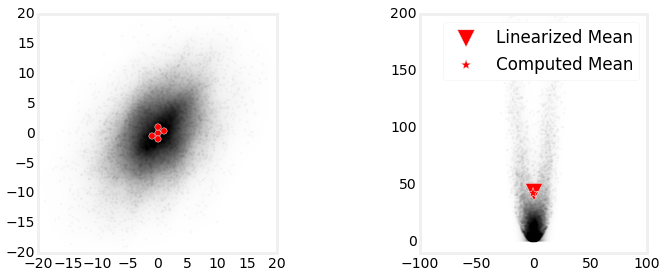I find this result remarkable. Using only 5 points we were able to compute the mean with amazing accuracy. The error in x is only 0.054, and the error in y is 0.408. In contrast, a linearized approach (used by the predominant EKF filter) gave an error of over 43 in y. I told you to ignore the code to generate the sigma points, but if you look at it you'll see that it has no knowledge of the nonlinear function, only of the mean and covariance of our initial distribution. The same 5 sigma points would be generated if we had a completely different nonlinear function.

I will admit to choosing a nonlinear function that makes the performance of the unscented tranform striking compared to the EKF. But the physical world is filled with very nonlinear behavior, and the UKF takes it in stride. You will see in the next chapter how more traditional techniques struggle with strong nonlinearities. This graph is the foundation of why I advise you to use the UKF or similar modern technique whenever possible.

The UKF belongs to a family of filters called sigma point filters. By the end of this chapter you will be prepared to do a literature search and learn about the various sigma point filters that have been invented.

## The Unscented Filter¶

Now we can present the entire Unscented Kalman filter algorithm. As discussed earlier assume that there is a function $f(x, dt)$ that performs the state transition for our filter - it predicts the next state given the current state. Also assume there is a measurement function $h(x)$ - it takes the current state and computes what measurement that state corresponds to. These are nonlinear forms of the $\mathbf{F}$ and $\mathbf{H}$ matrices used by the linear Kalman filter.

### Predict Step¶

As with the linear Kalman filter, the UKF's predict step computes the mean and covariance of the system for the next epoch using the process model. However, the computation is quite different.

First, we generate the weights and sigma points as specified above.

\begin{aligned} \mathcal{X} &= sigma\_function(\bf{\mu}, \bf{\Sigma}) \\ W &= weight\_function()\end{aligned}

We pass each sigma point through the function f. This projects the sigma points forward in time according to our process model.

$$\boldsymbol{\mathcal{Y}} = f(\boldsymbol{\chi})$$

Now we compute the predicted mean and covariance using the unscented transform on the transformed sigma points. I've dropped the subscript $i$ for readability.

\begin{aligned} \mathbf{\mu}^- &= \sum w^m\boldsymbol{\mathcal{Y}} \\ \mathbf{\Sigma}^- &= \sum w^c({\boldsymbol{\mathcal{Y}}-\bf{\mu}^-)(\boldsymbol{\mathcal{Y}}-\bf{\mu}^-)^\mathsf{T}} + \mathbf{Q} \end{aligned}

This computes the mean and covariance represented by the green ellipse above, and corresponds with the linear Kalman filter equations of

\begin{aligned} \mathbf{x}^- &= \mathbf{Fx}\\ \mathbf{P}^- &= \mathbf{FPF}^\mathsf{T}+\mathbf{Q} \end{aligned}

### Update Step¶

Now we can perform the update step of the filter. Recall that Kalman filters perform the update state in measurement space. So, the first thing we do is convert the sigma points from the predict step into measurements using the $h(x)$ function that you define.

$$\boldsymbol{\mathcal{Z}} = h(\boldsymbol{\mathcal{Y}})$$

Now we can compute the mean and covariance of these points using the unscented transform.

\begin{aligned} \mathbf{\mu}_z &= \sum w^m\boldsymbol{\mathcal{Z}} \\ \mathbf{P}_z &= \sum w^c{(\boldsymbol{\mathcal{Z}}-\mu^-)(\boldsymbol{\mathcal{Z}}-\mu^-)^\mathsf{T}} + \mathbf{R} \end{aligned}

The $z$ subscript denotes that these are the mean and covariance for the measurements.

All that is left is to compute the residual and Kalman gain. The residual is trivial to compute:

$$\mathbf{y} = \boldsymbol{\mathcal{Z}} - \boldsymbol{\mu}_z$$

The Kalman gain is not much worse. We have to compute the cross variance of the state and the measurements, which we state without proof to be:

$$\mathbf{P}_{xz} =\sum w^c(\boldsymbol{\chi}-\mu)(\boldsymbol{\mathcal{Z}}-\mathbf{\mu}_z)^\mathsf{T}$$

And then the Kalman gain is defined as

$$K = \mathbf{P}_{xz} \mathbf{P}_z^{-1}$$

Finally, we compute the new state estimate using the residual and Kalman gain:

$$\hat{\mathbf{x}} = \mathbf{x}^- + \mathbf{Ky}$$

and the new covariance is computed as:

$$\hat{\mathbf{P}} = \mathbf{\Sigma}^- - \mathbf{KP_z}\mathbf{K}^\mathsf{T}$$

This step contains a few equations you have to take on faith, but you should be able to see how they relate to the linear Kalman filter equations. We convert the mean and covariance into measurement space, add the measurement error into the measurement covariance, compute the residual and kalman gain, compute the new state estimate as the old estimate plus the residual times the Kalman gain, and then convert both back into state space. The linear algebra is slightly different from the linear Kalman filter, but the algorithm is the same.

## Using the UKF¶

We are now ready to consider implementing an unscented Kalman filter. All of the math is above is already implemented in FilterPy, and you are perhaps a bit lost at this point, so lets launch into solving some problems so you can gain confidence in how easy the UKF actually is. Later we will revisit how the UKF is implemented in Python.

Let's start by solving a problem you already know how to do. Although the UKF was designed for nonlinear problems, it works fine on linear problems. In fact, it is guaranteed to get the same result as the linear Kalman filter for linear problems. We will write a solver for the linear problem of tracking using a constant velocity model in 2D. This will allows us to focus on what is the same (and most is the same!) and what is different with the UKF.

To design a linear Kalman filter you need to design the $\bf{x}$, $\bf{F}$, $\bf{H}$, $\bf{R}$, and $\bf{Q}$ matrices. We have done this many times already so let me present a design to you without a lot of comment. We want a constant velocity model, so we can define $\bf{x}$ to be

$$\mathbf{x} = \begin{bmatrix}x & \dot{x} & y & \dot{y} \end{bmatrix}^\mathsf{T}$$

.

With this ordering of state variables we can define our state transition model to be

$$\mathbf{F} = \begin{bmatrix}1 & \Delta t & 0 & 0 \\ 0&1&0&0 \\ 0&0&1&\Delta t\\ 0&0&0&1 \end{bmatrix}$$

which implements the Newtonian equation

$$x_k = x_{k-1} + \dot{x}_{k-1}\Delta t$$

Our sensors provide position measurements but not velocity, so the measurement function is

$$\mathbf{H} = \begin{bmatrix}1&0&0&0 \\ 0&0&1&0 \end{bmatrix}$$

Let's say our sensor gives positions in meters with an error of $1\sigma=0.3$ meters in both the x and y coordinates. This gives us a measurement noise matrix of

$$\mathbf{R} = \begin{bmatrix}0.3^2 &0\\0 & 0.3^2\end{bmatrix}$$

Finally, let's assume that the process noise can be represented by the discrete white noise model - that is, that over each time period the acceleration is constant. We can use FilterPy's Q_discrete_white_noise() method to create this matrix for us, but for review the matrix is

$$\mathbf{Q} = \begin{bmatrix} \frac{1}{4}\Delta t^4 & \frac{1}{2}\Delta t^3 \\ \frac{1}{2}\Delta t^3 & \Delta t^2\end{bmatrix} \sigma^2$$

Our implementation might look like this:

In :
from filterpy.kalman import KalmanFilter
from filterpy.common import Q_discrete_white_noise
from numpy import random
from numpy.random import randn

sigma_x, sigma_y = .3, .3
dt = 1.0

random.seed(1234)
kf = KalmanFilter(4, 2)
kf.x = np.array([0., 0., 0., 0.])
kf.R = np.diag([sigma_x**2, sigma_y**2])
kf.F = np.array([[1, dt, 0, 0],
[0, 1, 0, 0],
[0, 0, 1, dt],
[0, 0, 0, 1]])
kf.H = np.array([[1, 0, 0, 0],
[0, 0, 1, 0]])

kf.Q[0:2, 0:2] = Q_discrete_white_noise(2, dt=1, var=0.02)
kf.Q[2:4, 2:4] = Q_discrete_white_noise(2, dt=1, var=0.02)

zs = [np.array([i + randn()*sigma_x, i + randn()*sigma_y]) for i in range(100)]
xs, _, _, _ = kf.batch_filter(zs)
plt.plot(xs[:, 0], xs[:, 2]);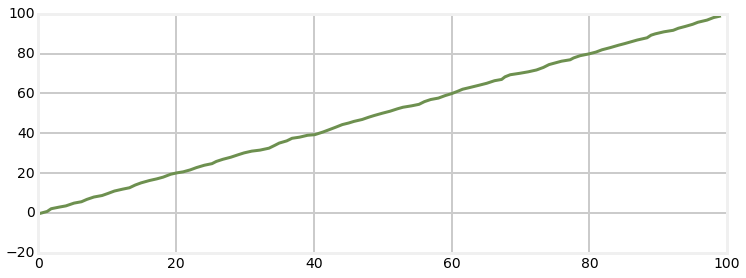This should hold no surprises for you. Now let's implement this filter as an Unscented Kalman filter. Again, this is purely for educational purposes; using a UKF for a linear filter confers no benefit.

The equations of the UKF are implemented for you with the FilterPy class UnscentedKalmanFilter; all you have to do is specify the matrices and the nonlinear functions f(x) and h(x). f(x) implements the state transition function that is implemented by the matrix $\mathbf{F}$ in the linear filter, and h(x) implements the measurement function implemented with the matrix $\mathbf{H}$. In nonlinear problems these functions are nonlinear, so we cannot use matrices to specify them.

For our linear problem we can define these functions to implement the linear equations. The function f(x) takes the signature def f(x,dt) and h(x) takes the signature def h(x). Below is a reasonable implementation of these two functions. Each is expected to return a 1-D NumPy array with the result.

In :
def f_cv(x, dt):
""" state transition function for a constant velocity aircraft"""

F = np.array([[1, dt, 0,  0],
[0,  1, 0,  0],
[0,  0, 1, dt],
[0,  0, 0,  1]])
return np.dot(F, x)

def h_cv(x):
return np.array([x, x])


You need to tell the filter how to compute the sigma points and weights. We gave the Van der Merwe's scaled unscented transform version above, but there are many different choices. FilterPy uses a SigmaPoints class which must implement two methods:

def sigma_points(self, x, P)
def weights(self)



FilterPy provides the class MerweScaledSigmaPoints, which implements the sigma point algorithm in this chapter.

Other than these two functions, everything else is nearly the same. When you create the UKF you will pass in the two functions and sigma point class object like so:

from filterpy.kalman import MerweScaledSigmaPoints
from filterpy.kalman import UnscentedKalmanFilter as UKF

points = MerweScaledSigmaPoints(n=4, alpha=.1, beta=2., kappa=-1)
ukf = UKF(dim_x=4, dim_z=2, fx=f_cv, hx=h_cv, dt=dt, points=points)

The rest of the code is the same as for the linear kalman filter. Let's just implement it! I'll use the same measurements as used by the linear Kalman filter, and compute the standard deviation of the difference between the two solution. I will use the batch_filter method to keep the code succinct; for clarity I put the equivalent iterative loop in a comment. As with all the filters we have done to date you ju

In :
from filterpy.kalman import MerweScaledSigmaPoints
from filterpy.kalman import UnscentedKalmanFilter as UKF

import numpy as np

sigmas = MerweScaledSigmaPoints(4, alpha=.1, beta=2., kappa=1.)
ukf = UKF(dim_x=4, dim_z=2, fx=f_cv, hx=h_cv, dt=dt, points=sigmas)
ukf.x = np.array([0., 0., 0., 0.])
ukf.R = np.diag([0.09, 0.09])
ukf.Q[0:2, 0:2] = Q_discrete_white_noise(2, dt=1, var=0.02)
ukf.Q[2:4, 2:4] = Q_discrete_white_noise(2, dt=1, var=0.02)

uxs = []
for z in zs:
ukf.predict()
ukf.update(z)
uxs.append(ukf.x.copy())
uxs = np.array(uxs)

plt.plot(uxs[:, 0], uxs[:, 2])
print('UKF standard deviation {:.3f}'.format(np.std(uxs - xs)))

UKF standard deviation 0.013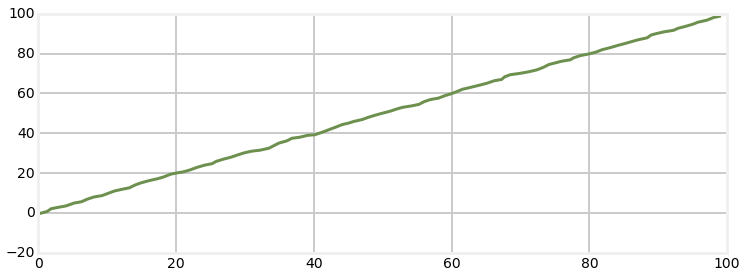This gave me a standard deviation 0f 0.013 which is quite small.

You can see that there is not much difference in the implementation of the UKF vs linear Kalman filter. We merely replace $\mathbf{F}$ with the function f(x), and the matrix $\mathbf{H}$ with the function h(x). The rest of the theory and implementation remains the same. Well, of course under the hood the FilterPy implementation is quite different than the Kalman filter code, but from a designer's point of view the problem formulation and filter design is very similar.

## Tracking a Flying Airplane¶

### First Attempt¶

Let's tackle our first nonlinear problem. To minimize the number of things that change I will keep the problem formulation very similar to the linear tracking problem above. For this problem we will write a filter to track a flying airplane using a stationary radar as the sensor. To keep the problem as close to the previous one as possible we will track in two dimensions, not three. We will track one dimension on the ground and the altitude of the aircraft. The second dimension on the ground adds no difficulty or different information, so we can do this with no loss of generality.

For this example we want to take the slant range measurement from the radar and compute the horizontal position (distance of aircraft from the radar measured over the ground) and altitude of the aircraft, as in the diagram below.

In :
import ekf_internal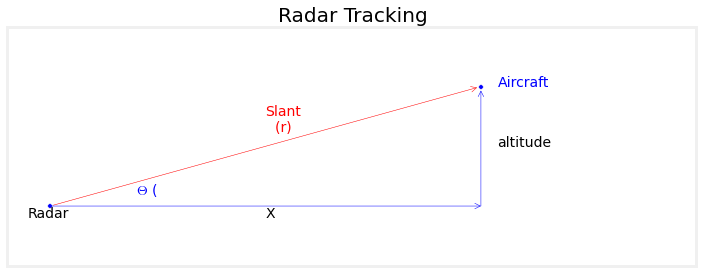We will assume that the aircraft is flying at a constant altitude, so a three variable state vector will work.

$$\mathbf{x} = \begin{bmatrix}distance \\velocity\\ altitude\end{bmatrix}= \begin{bmatrix}x_{pos} \\x_{vel}\\ x_{alt}\end{bmatrix}$$

Our state transition function is linear

$$\mathbf{x}^- = \begin{bmatrix} 1 & \Delta t & 0 \\ 0& 1& 0 \\ 0&0&1\end{bmatrix} \begin{bmatrix}x_{pos} \\x_{vel}\\ x_{alt}\end{bmatrix}$$

and we can implement that very much like we did for the previous problem.

In :
def f_radar(x, dt):
""" state transition function for a constant velocity aircraft
with state vector [x, velocity, altitude]'"""

F = np.array([[1, dt, 0],
[0,  1, 0],
[0,  0, 1]], dtype=float)
return np.dot(F, x)


The next step is to design the measurement function. As in the linear Kalman filter the measurement function converts the filter's state into a measurement. So for this problem we need the position and velocity of the aircraft and convert it to the bearing and range from the radar station.

If we represent the position of the radar station with an (x,y) coordinate computation of the range and bearing is straightforward. To compute the range we use the Pythagorean theorem:

$$range = \sqrt{(x_{ac} - x_{radar})^2 + (z_{ac} - z_{radar})^2}$$

To compute the bearing we need to use the arctangent function.

$$bearing = \tan^{-1}{\frac{z_{ac} - z_{radar}}{x_{ac} - x_{radar}}}$$

As with the state transition function we need to define a Python function to compute this for the filter. I'll take advantage of the fact that a function can own a variable to store the radar's position.

In :
def h_radar(x):
slant_range = math.sqrt(dx**2 + dz**2)
bearing = math.atan2(dz, dx)
return slant_range, bearing



Important Note: There is a nonlinearity that we are not considering, the fact that angles are modular. Kalman filters operate by computing the differences between measurements. The difference between $359^\circ$ and $1^\circ$ is $2^\circ$, but a subtraction of the two values, as implemented by the filter, yields a value of $358^\circ$. This is exacerbated by the UKF which computes sums of weighted values in the unscented transform. For now we will place our sensors and targets in positions that avoid these nonlinear regions. Later in the chapter I will show you how to handle this problem.

We need to simulate the Radar station and the movement of the aircraft. By now this should be second nature for you, so I will present the code without much discussion.

In :
from numpy.linalg import norm
from math import atan2

def __init__(self, pos, range_std, bearing_std):
self.pos = np.asarray(pos)

self.range_std = range_std
self.bearing_std = bearing_std

""" Returns actual range and bearing to aircraft as tuple.
"""

diff = np.subtract(ac_pos, self.pos)
rng = norm(diff)
brg = atan2(diff, diff)
return rng, brg

""" Compute range and bearing to aircraft with simulated noise"""

rng += randn() * self.range_std
brg += randn() * self.bearing_std
return rng, brg

class ACSim(object):

def __init__(self, pos, vel, vel_std):
self.pos = np.asarray(pos, dtype=float)
self.vel = np.asarray(vel, dtype=float)
self.vel_std = vel_std

def update(self, dt):
""" compute next position. Incorporates random variation in
velocity. Returns new position."""

vel = self.vel*dt + (randn() * self.vel_std) * dt
self.pos += vel

return self.pos


Now let's put it all together. A military grade radar system can achieve 1 meter RMS range accuracy, and 1 mrad RMS for bearing . We will assume a more modest 5 meter range accuracy, and 0.5$^\circ$ angular accuracy as this provides a more challenging data set for the filter.

I'll start with the aircraft positioned directly over the radar station, flying away from it at 100 m/s. A typical radar might update only once every 12 seconds and so we will use that for our update period.

In :
import book_plots
from filterpy.common import Q_discrete_white_noise
import math

dt = 12. # 12 seconds between readings

range_std = 5 # meters

ac_pos = (0., 1000.)
ac_vel = (100., 0.)

points = MerweScaledSigmaPoints(n=3, alpha=.1, beta=2., kappa=0.)

kf.Q[0:2, 0:2] = Q_discrete_white_noise(2, dt=dt, var=0.1)
kf.Q[2, 2] = 0.1

kf.R = np.diag([range_std**2, bearing_std**2])
kf.x = np.array([0., 90., 1100.])
kf.P = np.diag([300**2, 30**2, 150**2])

ac = ACSim(ac_pos, (100, 0), 0.02)

random.seed(200)

t = np.arange(0, 360 + dt, dt)
n = len(t)

xs = []
for i in range(len(t)):
ac.update(dt)
kf.predict()
kf.update([r, r])
xs.append(kf.x)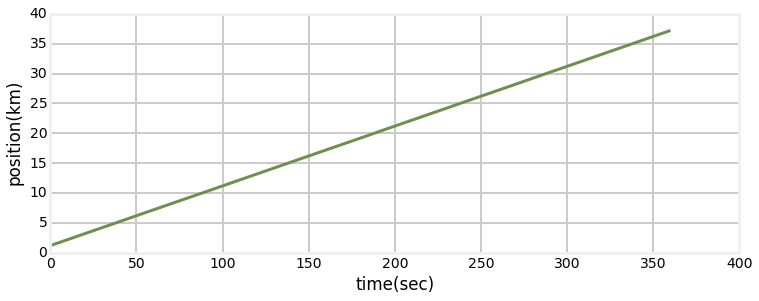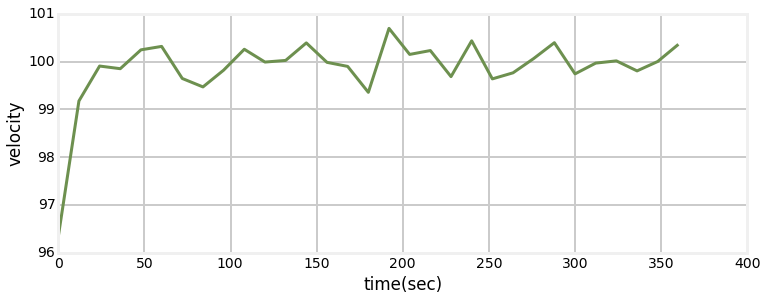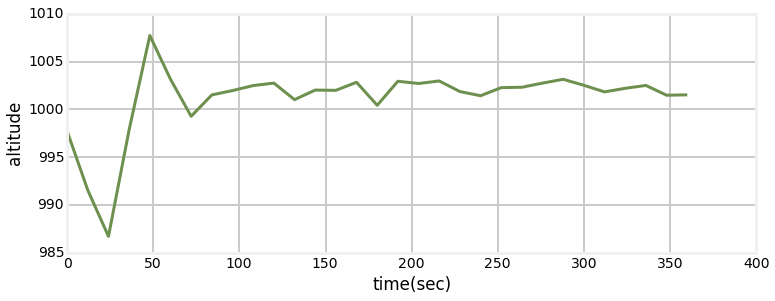This may or may not impress you, but it impresses me! In the Extended Kalman filter chapter we will solve the same problem, but it will take significant amounts of mathematics to handle the same problem.

### Tracking Manuevering Aircraft¶

The previous example produced extremely good results, but it also relied on an assumption of an aircraft flying at a constant speed with no change in altitude. I will relax that assumption by allowing the aircraft to change altitude. First, let's see the performance of the previous code if the aircraft starts climbing after one minute.

In :
# reset aircraft position
kf.x = np.array([0., 90., 1100.])
kf.P = np.diag([300**2, 30**2, 150**2])
ac = ACSim(ac_pos, (100, 0), 0.02)

random.seed(200)

t = np.arange(0, 360 + dt, dt)
n = len(t)

xs = []
for i in t:
if i >= 60:
ac.vel = 300/60 # 300 meters/minute climb
ac.update(dt)
kf.predict()
kf.update([r, r])
xs.append(kf.x)

print('Actual altitude: {:.1f}'.format(ac.pos))
print('UKF altitude   : {:.1f}'.format(xs[-1]))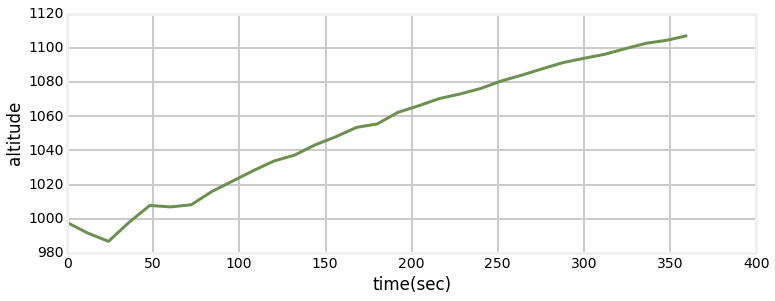Actual altitude: 2561.9
UKF altitude   : 1107.2


the performance is terrible as the filter is completely unable to track the changing altitude. What do we have to change to allow the filter to track the aircraft?

I hope you answered add climb rate to the state, like so:

$$\mathbf{x} = \begin{bmatrix}distance \\velocity\\ altitude \\ climb rate\end{bmatrix}= \begin{bmatrix}x \\\dot{x}\\ z \\ \dot{z}\end{bmatrix}$$

This requires the following change to the state transition function, which is still linear.

$$\mathbf{x}^- = \begin{bmatrix} 1 & \Delta t & 0 &0 \\ 0& 1& 0 &0\\ 0&0&1&dt \\ 0&0&0&1\end{bmatrix} \begin{bmatrix}x \\\dot{x}\\ z\\ \dot{z}\end{bmatrix}$$

The measurement function stays the same, but we will have to alter Q to account for the state dimensionality change.

In :
def f_cv_radar(x, dt):
""" state transition function for a constant velocity aircraft"""

F = np.array([[1, dt, 0, 0],
[0,  1, 0, 0],
[0,  0, 1, dt],
[0,  0, 0, 1]], dtype=float)
return np.dot(F, x)

ac = ACSim(ac_pos, (100, 0), 0.02)

points = MerweScaledSigmaPoints(n=4, alpha=.1, beta=2., kappa=-1.)

kf.Q[0:2, 0:2] = Q_discrete_white_noise(2, dt=dt, var=0.1)
kf.Q[2:4, 2:4] = Q_discrete_white_noise(2, dt=dt, var=0.1)

kf.R = np.diag([range_std**2, bearing_std**2])
kf.x = np.array([0., 90., 1100., 0.])
kf.P = np.diag([300**2, 3**2, 150**2, 3**2])

random.seed(200)

t = np.arange(0, 360 + dt, dt)
n = len(t)

xs = []
for i in t:
if i >= 60:
ac.vel = 300/60 # 300 meters/minute climb
ac.update(dt)
kf.predict()
kf.update([r, r])
xs.append(kf.x)

print('Actual altitude: {:.1f}'.format(ac.pos))
print('UKF altitude   : {:.1f}'.format(xs[-1]))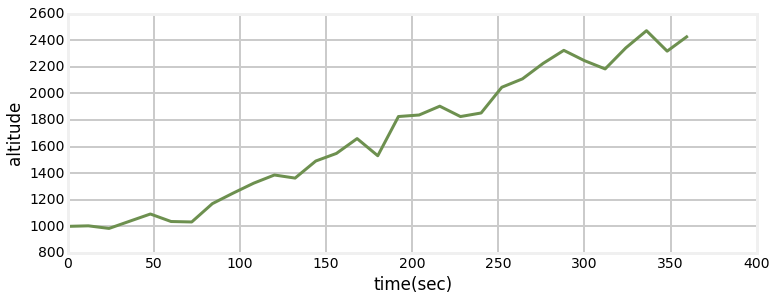Actual altitude: 2561.9
UKF altitude   : 2432.9


We can see that a significant amount of noise has been introduced into the altitude, but we are now accurately tracking the altitude change.

### Sensor Fusion¶

Now let's consider a simple example of sensor fusion. Suppose we have some type of Doppler system that produces a velocity estimate with 2 m/s RMS accuracy. I say "some type" because as with the radar I am not trying to teach you how to create an accurate filter for a Doppler system, where you have to account for the signal to noise ratio, atmospheric effects, the geometry of the system, and so on.

The accuracy of the radar system in the last examples allowed us to estimate velocities to within a m/s or so, so we have to degrade that accuracy to be able to easily see the effect of the sensor fusion. Let's change the range error to 500 meters and then compute the standard deviation of the computed velocity. I'll skip the first several measurements because the filter is converging during that time, causing artificially large deviations.

In :
range_std = 500.
bearing_std = math.degrees(0.5)
ac = ACSim(ac_pos, (100, 0), 0.02)

points = MerweScaledSigmaPoints(n=4, alpha=.1, beta=2., kappa=-1.)

kf.Q[0:2, 0:2] = Q_discrete_white_noise(2, dt=dt, var=0.1)
kf.Q[2:4, 2:4] = Q_discrete_white_noise(2, dt=dt, var=0.1)

kf.R = np.diag([range_std**2, bearing_std**2])
kf.x = np.array([0., 90., 1100., 0.])
kf.P = np.diag([300**2, 3**2, 150**2, 3**2])

random.seed(200)

t = np.arange(0, 360 + dt, dt)
n = len(t)

xs = []
for i in t:
ac.update(dt)
kf.predict()
kf.update([r, r])
xs.append(kf.x)

xs = np.asarray(xs)
print('Velocity standard deviation {:.1f} m/s'.format(np.std(xs[10:, 1])))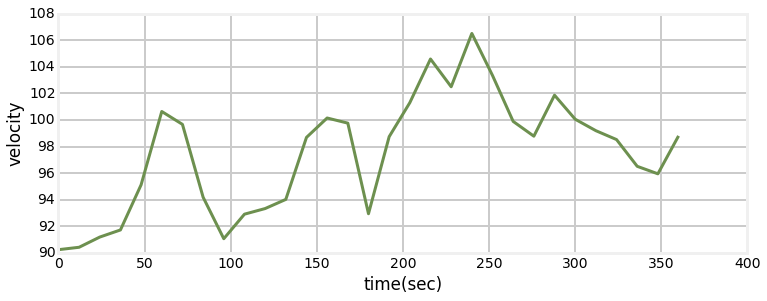Velocity standard deviation 3.4 m/s

In :
def h_vel(x):
slant_range = math.sqrt(dx**2 + dz**2)
bearing = math.atan2(dz, dx)
return slant_range, bearing, x, x

range_std = 500.
bearing_std = math.degrees(0.5)
vel_std = 2.

ac = ACSim(ac_pos, (100, 0), 0.02)
points = MerweScaledSigmaPoints(n=4, alpha=.2, beta=2., kappa=-1.)
kf = UKF(4, 4, dt, fx=f_cv_radar, hx=h_vel, points=points)

kf.Q[0:2, 0:2] = Q_discrete_white_noise(2, dt=dt, var=0.1)
kf.Q[2:4, 2:4] = Q_discrete_white_noise(2, dt=dt, var=0.1)

kf.R = np.diag([range_std**2, bearing_std**2, vel_std**2, vel_std**2])
kf.x = np.array([0., 90., 1100., 0.])
kf.P = np.diag([300**2, 3**2, 150**2, 3**2])

random.seed(200)

t = np.arange(0, 360 + dt, dt)
n = len(t)

xs = []
for i in t:
ac.update(dt)
vx = ac.vel + randn()*vel_std
vz = ac.vel + randn()*vel_std
kf.predict()
kf.update([r, r, vx, vz])
xs.append(kf.x)
xs = np.asarray(xs)
print('Velocity standard deviation {:.1f} m/s'.format(np.std(xs[10:,1])))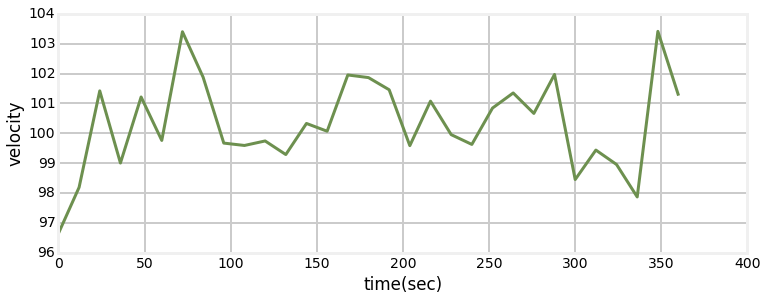Velocity standard deviation 1.3 m/s


By incorporating the velocity sensor we were able to reduce the standard deviation from 3.5 m/s to 1.3 m/s.

Sensor fusion is a large topic, and this is a rather simplistic implementation. In a typical navigation problem we have sensors that provide complementary information. For example, a GPS might provide somewhat accurate position updates once a second with poor velocity estimation while an inertial system might provide very accurate velocity updates at 50Hz but terrible position estimates. The strengths and weaknesses of each sensor are orthogonal to each other. This leads to something called the Complementary filter, which uses the high update rate of the inertial sensor with the position accurate but slow estimates of the GPS to produce very high rate yet very accurate position and velocity estimates. This will be the topic of a future chapter.

### Multiple Position Sensors¶

The last sensor fusion problem was somewhat a toy example due to the existence of techniques like the Complementary filter. Let's tackle a problem that is not so toy-like. Before the advent of GPS ships and aircraft navigated via various range and bearing systems such as VOR, LORAN, TACAN, DME, and so on. I do not intend to cover the intricacies of these systems - Wikipedia will fill the basics if you are interested. In general these systems are beacons that allow you to extract either the range, bearing, or range and bearing to the beacon. For example, an aircraft might have two VOR receivers. The pilot tunes each receiver to a different VOR station. Each VOR receiver displays what is called the "radial" - the direction from the VOR station on the ground to the aircraft. Using a chart they can extend these two radials - the intersection point is the position of the aircraft.

That is a very manual and low accuracy approach. We can use a Kalman filter to filter the data and produce far more accurate position estimates. Let's work through that.

The problem is as follows. Assume we have two sensors, each which provides a bearing only measurement to the target, as in the chart below. In the chart the width of the circle is intended to denote a different amount of sensor noise.

In :
ukf_internal.show_two_sensor_bearing()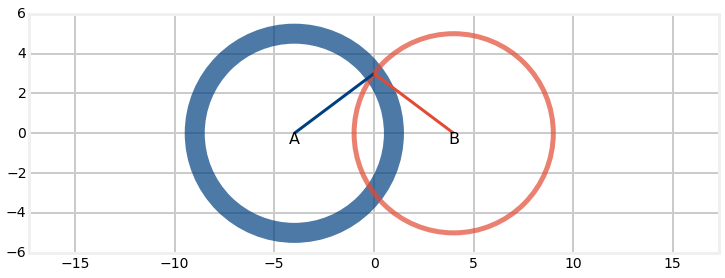We can compute the bearing between a sensor and the target as follows:

def bearing(sensor, target):
return math.atan2(target - sensor, target - sensor)

So our filter will need to receive a vector of 2 measurements during each update, one for each sensor. We can implement that as:

def measurement(A_pos, B_pos, pos):
angle_a = bearing(A_pos, pos)
angle_b = bearing(B_pos, pos)
return [angle_a, angle_b]

The design of the measurement function and state transition function can remain the same as nothing has changed that would affect them.

In :
from filterpy.kalman import UnscentedKalmanFilter as UKF
from filterpy.common import Q_discrete_white_noise

sa_pos = [-400, 0]
sb_pos = [400, 0]

def bearing(sensor, target_pos):
return math.atan2(target_pos - sensor, target_pos - sensor)

def measurement(A_pos, B_pos, pos):
angle_a = bearing(A_pos, pos)
angle_b = bearing(B_pos, pos)
return [angle_a, angle_b]

def fx_VOR(x, dt):
x += x
x += x
return x

def hx_VOR(x):
# measurement to A
pos = (x, x)
return measurement(sa_pos, sb_pos, pos)

def moving_target_filter(target_pos, std_noise, Q, dt=0.1, kappa=0.0):
points = MerweScaledSigmaPoints(n=4, alpha=.1, beta=2., kappa=kappa)
f = UKF(dim_x=4, dim_z=2, dt=dt, hx=hx_VOR, fx=fx_VOR, points=points)
f.x = np.array([target_pos, 1., target_pos, 1.])

q = Q_discrete_white_noise(2, dt, Q)
f.Q[0:2, 0:2] = q
f.Q[2:4, 2:4] = q

f.R *= std_noise**2
f.P *= 1000

return f

def plot_straight_line_target(f, std_noise):
xs = []
txs = []

for i in range(300):
target_pos += 1 + randn()*0.0001
target_pos += 1 + randn()*0.0001
txs.append((target_pos, target_pos))

z = measurement(sa_pos, sb_pos, target_pos)
z += randn() * std_noise
z += randn() * std_noise

f.predict()
f.update(z)
xs.append(f.x)

xs = np.asarray(xs)
txs = np.asarray(txs)

plt.plot(xs[:, 0], xs[:, 2])
plt.plot(txs[:, 0], txs[:, 1])
plt.show()

np.random.seed(123)
target_pos = [100, 200]

f = moving_target_filter(target_pos, std_noise, Q=1.0)
plot_straight_line_target(f, std_noise)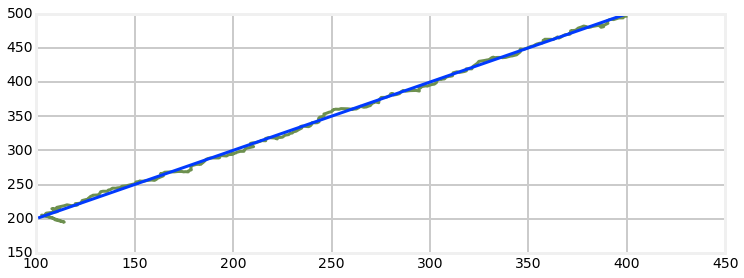This looks quite good to me. There is a very large error at the beginning of the track, but the filter is able to settle down and start producing good data.

Let's revisit the nonlinearity of the angles. I will position the target between the two sensors with the same y-coordinate. This will cause a nonlinearity in the computation of the sigma means and the residuals because the mean angle will be near zero. As the angle goes below 0 the measurement function will compute a large positive angle of around $2\pi$. The residual between the prediction and measurement will thus be very large, nearly $2\pi$ instead of nearly 0. This makes it impossible for the filter to perform accurately, as seen in the example below.

In :
target_pos = [0, 0]
f = moving_target_filter(target_pos, std_noise, Q=1.0)
plot_straight_line_target(f, std_noise)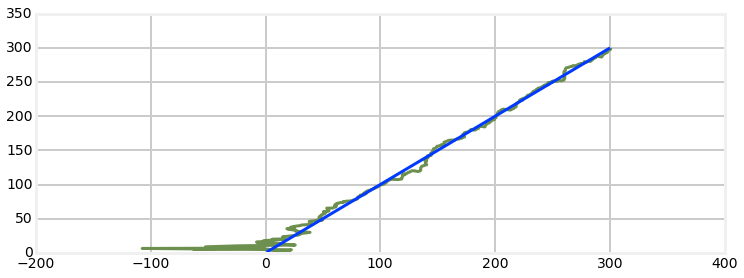This is untenable behavior for a real world filter. FilterPy's UKF code allows you to provide it a function to compute the residuals in cases of nonlinear behavior like this, but this requires more knowledge about FilterPy's implementation than we yet process.

Finally, let's discuss the sensor fusion method that we used. We used the measurement from each bearing and had the Kalman filter's equations compute the world position from the measurements. This is equivalent to doing a weighted least squares solution. In the Kalman Filter Math chapter we discussed the limited accuracy of such a scheme and introduced an Iterative Least Squares (ILS) algorithm to produce greater accuracy. If you wanted to use that scheme you would write an ILS algorithm to solve the geometry of your problem, and pass the result of that calculation into the update() method as the measurement to be filtered. This imposes on you the need to compute the correct noise matrix for this computed positions, which may not be trivial. Perhaps in a later release of this book I will develop an example, but regardless it will take you a significant amount of research and experiment to design the best method for your application. For example, the ILS is probably the most common algorithm used to turn GPS pseudoranges into a position, but the literature discusses a number of alternatives. Sometimes there are faster methods, sometimes the iterative method does not converge, or does not converge fast enough, or requires too much computation for an embedded system.

## Exercise: Track a target moving in a circle¶

Change the simulated target movement to move in a circle. Avoid angular nonlinearities by putting the sensors well outside the movement range of the target, and avoid the angles $0^\circ$ and $180^\circ$.

In :
# your solution here


### Solution¶

We have a few choices here. First, if we know the movement of the target we can design our filter's state transition function so that it correctly predicts the circular movement. For example, suppose we were tracking a boat race optically - we would want to take track shape into account with our filter. However, in this chapter we have not been talking about such constrained behavior, so I will not build knowledge of the movement into the filter. So my implementation looks like this.

In :
def plot_circular_target(kf, std_noise, target_pos):
xs = []
txs = []
for i in range(300):
txs.append((target_pos, target_pos))

z = measurement(sa_pos, sb_pos, target_pos)
z += randn() * std_noise
z += randn() * std_noise

kf.predict()
kf.update(z)
xs.append(kf.x)

xs = np.asarray(xs)
txs = np.asarray(txs)

plt.plot(xs[:, 0], xs[:, 2])
plt.plot(txs[: ,0], txs[:, 1], linestyle='-.')
plt.axis('equal')
plt.show()

sa_pos = [-240, 200]
sb_pos = [240, 200]

In :
np.random.seed(12283)
target_pos = [0, 0]
f = moving_target_filter(target_pos, std_noise, Q=1.1)
plot_circular_target(f, std_noise, target_pos)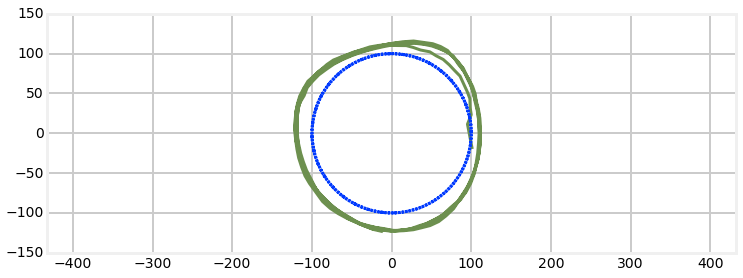### Discussion¶

The filter tracks the movement of the target, but never really converges on the track. This is because the filter is modeling a constant velocity target, but the target is anything but constant velocity. As mentioned above we could model the circular behavior by defining the fx() function, but then we would have problems when the target is not moving in a circle. Instead, lets tell the filter we are are less sure about our process model by making $\mathbf{Q}$ larger. Here I have increased the variance from 0.1 to 1.0

In :
np.random.seed(12283)
cf = moving_target_filter(target_pos, std_noise, Q=10.)
target_pos = [0, 0]
plot_circular_target(cf, std_noise, target_pos)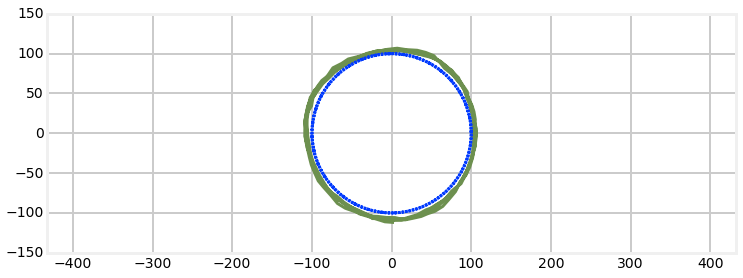The convergence is not perfect, but it is far better.

## Exercise: Sensor Position Effects¶

Is the behavior of the filter invariant for any sensor position? Find a sensor position that produces bad filter behavior.

In :
# your answer here


### Solution¶

We have already discussed the problem of angles being modal, so causing that problem by putting the sensors at y=0 would be a trivial solution. However, let's be more subtle than that. We want to create a situation where there are an infinite number of solutions for the sensor readings. We can achieve that whenever the target lies on the straight line between the two sensors. In that case there is no triangulation possible and there is no unique solution. My solution is as follows.

In :
std_noise = math.radians(0.5)
sa_pos = [-200, 200]
sb_pos = [200, -200]
plt.scatter(*sa_pos, s=200)
plt.scatter(*sb_pos, s=200)
target_pos = [0, 0]
cf = moving_target_filter(target_pos, std_noise, Q=10.)
plot_circular_target(cf, std_noise, target_pos)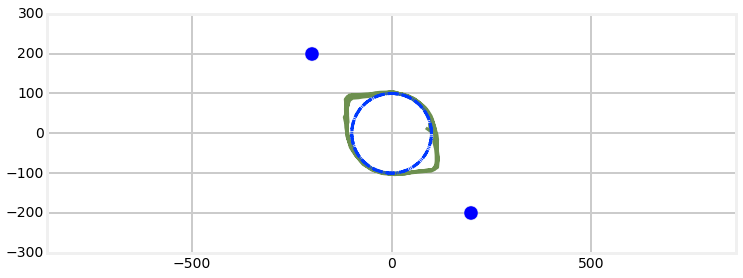I put the sensors at the upper left hand side and lower right hand side of the target's movement. We can see how the filter diverges when the target enters the region directly between the two sensors. The state transition always predicts that the target is moving in a straight line. When the target is between the two sensors this straight line movement is well described the bearing measurements from the two sensors so the filter estimate starts to approximate a straight line.

## Exercise: Compute Position Errors¶

The position errors of the filter vary depending on how far away the target is from a sensor. Write a function that computes the distance error due to a bearing error.

In :
# your solution here


### Solution¶

Basic trigonometry gives us this answer.

In :
def distance_error(target_distance, angle_error):
x = 1 - math.cos(angle_error)
y = math.sin(angle_error)
return target_distance*(x**2 + y**2)**.5


Error of 1 degree at 100km is 1.75km